###### pandas groupby: split-apply-combine,
In :
`import pandas as pd`
`import numpy as np`
`import matplotlib.pyplot as plt`
`np.random.seed(99)`
In :
`df = pd.DataFrame({'key1': ['a', 'a', 'b', 'b', 'a'],`
`                  'key2': ['one', 'two', 'one', 'two', 'one'],`
`                  'data1': np.random.randn(5),`
`                  'data2': np.random.randn(5)})`
`df`
Out:

data1data2key1key2
0-0.142359-0.069031aone
12.0572220.755180atwo
20.2832620.825647bone
31.329812-0.113069btwo
4-0.154622-2.367838aone
In :
`# groupby will return a series of two elements tuples; differences:`
`for gb in df.groupby('key1'):`
`    print gb`
`    print '-------------------'`
`print '------------------This is the split line----------------------'`
`for name, group in df.groupby(['key1']):`
`    print name`
`    print '----------------------'`
`    print group`
`print '------------------This is the split line----------------------'`
`for name, group in df.groupby(['key1', 'key2']):`
`    print name`
`    print '----------------------'`
`    print group`
`    print type(group)`
`('a',       data1     data2 key1 key20 -0.142359 -0.069031    a  one1  2.057222  0.755180    a  two4 -0.154622 -2.367838    a  one)-------------------('b',       data1     data2 key1 key22  0.283262  0.825647    b  one3  1.329812 -0.113069    b  two)-------------------------------------This is the split line----------------------a----------------------      data1     data2 key1 key20 -0.142359 -0.069031    a  one1  2.057222  0.755180    a  two4 -0.154622 -2.367838    a  oneb----------------------      data1     data2 key1 key22  0.283262  0.825647    b  one3  1.329812 -0.113069    b  two------------------This is the split line----------------------('a', 'one')----------------------      data1     data2 key1 key20 -0.142359 -0.069031    a  one4 -0.154622 -2.367838    a  one<class 'pandas.core.frame.DataFrame'>('a', 'two')----------------------      data1    data2 key1 key21  2.057222  0.75518    a  two<class 'pandas.core.frame.DataFrame'>('b', 'one')----------------------      data1     data2 key1 key22  0.283262  0.825647    b  one<class 'pandas.core.frame.DataFrame'>('b', 'two')----------------------      data1     data2 key1 key23  1.329812 -0.113069    b  two<class 'pandas.core.frame.DataFrame'>`
In :
`# change groupby output to dict`
`pieces = dict(list(df.groupby('key1')))`
`print pieces`
`{'a':       data1     data2 key1 key20 -0.142359 -0.069031    a  one1  2.057222  0.755180    a  two4 -0.154622 -2.367838    a  one, 'b':       data1     data2 key1 key22  0.283262  0.825647    b  one3  1.329812 -0.113069    b  two}`
In :
`# groupby througn dict or series (usually for columns)`
`np.random.seed(9999)`
`people = pd.DataFrame(np.random.randn(5, 5),`
`                      columns = list('abcde'),`
`                      index = ['Joe', 'Steve', 'Wes', 'Jim', 'Travis'])`
`people.ix[2:3, ['b', 'c']] = np.nan`
`mapping = {'a': 'red', 'b': 'red', 'c': 'blue', 'd': 'blue', 'e': 'red', 'f': 'orange'}`
`by_column = people.groupby(mapping, axis = 1)`
`by_column.sum()`
Out:

bluered
Joe-0.440531-0.278096
Steve0.8547861.354338
Wes-1.646232-1.021736
Jim-2.071417-1.432511
Travis1.853391-1.253057
In :
`# groupby througn index level(or column level)`
`np.random.seed(9999)`
`columns = pd.MultiIndex.from_arrays([['us', 'us', 'us', 'jp', 'jp'], [1, 3, 5, 1, 3]], names = ['cty', 'tenor'])`
`hier_df = pd.DataFrame(np.random.randn(4, 5), columns = columns)`
`print hier_df`
`print '-----------------------'`
`hier_df.groupby(level = 'cty', axis = 1).count()`
`cty          us                            jp          tenor         1         3         5         1         30     -0.515039  0.591897  0.047873 -0.488405 -0.3549531     -0.391813  1.864261 -1.371490  2.226275 -0.1181102      0.119848  0.182599 -0.657739 -1.646232 -1.1415843     -1.439089  0.744992 -1.739220 -0.332197 -0.738413-----------------------`
Out:
ctyjpus
023
123
223
323
In :
`# use different function for different columns`
`def peak_to_peak(arr):`
`    return arr.max() - arr.min()`
`tips = pd.read_csv('bookdata/ch08/tips.csv')`
`tips['tip_pct'] = tips['tip'] / tips['total_bill']`
`grouped = tips.groupby(['sex', 'smoker'])`
`grouped_pct = grouped['tip_pct']`
` `
`print grouped_pct.agg(['mean', 'std', peak_to_peak])`
` `
`print '------------------- rename columns ----------------------'`
`print grouped_pct.agg([('foo', 'mean'), ('bar', 'std')])`
` `
`print '------------------- function on all columns ----------------------'`
`functions = ['count', 'mean', 'max']`
`print grouped['tip_pct', 'total_bill'].agg(functions)`
` `
`print '------------------- rename columns ----------------------'`
`ftuples = [('Im Mean', 'mean'), ('Im var', np.var)]`
`print grouped['tip_pct', 'total_bill'].agg(ftuples)`
` `
`print '------------------- diff funs on diff cols ----------------------'`
`print grouped.agg({'tip': [np.max, 'mean'], 'size': 'sum'})`
`                   mean       std  peak_to_peaksex    smoker                                  Female No      0.156921  0.036421      0.195876       Yes     0.182150  0.071595      0.360233Male   No      0.160669  0.041849      0.220186       Yes     0.152771  0.090588      0.674707------------------- rename columns ----------------------                    foo       barsex    smoker                    Female No      0.156921  0.036421       Yes     0.182150  0.071595Male   No      0.160669  0.041849       Yes     0.152771  0.090588------------------- function on all columns ----------------------               tip_pct                      total_bill                                   count      mean       max       count       mean    maxsex    smoker                                                           Female No           54  0.156921  0.252672          54  18.105185  35.83       Yes          33  0.182150  0.416667          33  17.977879  44.30Male   No           97  0.160669  0.291990          97  19.791237  48.33       Yes          60  0.152771  0.710345          60  22.284500  50.81------------------- rename columns ----------------------                tip_pct            total_bill                           Im Mean    Im var     Im Mean     Im varsex    smoker                                           Female No      0.156921  0.001327   18.105185  53.092422       Yes     0.182150  0.005126   17.977879  84.451517Male   No      0.160669  0.001751   19.791237  76.152961       Yes     0.152771  0.008206   22.284500  98.244673------------------- diff funs on diff cols ----------------------                tip            size               amax      mean   sumsex    smoker                      Female No       5.2  2.773519   140       Yes      6.5  2.931515    74Male   No       9.0  3.113402   263       Yes     10.0  3.051167   150`
In :
`# apply function on each piece of groupby`
`def top(df, n=5, columns = 'tip_pct'):`
`    return df.sort_index(by = columns)[-n:]`
`tips.groupby(['smoker', 'day']).apply(top, n=2, columns = 'total_bill')`
Out:

total_billtipsexsmokerdaytimesizetip_pct
smokerday

NoFri9122.493.50MaleNoFriDinner20.155625
9422.753.25FemaleNoFriDinner20.142857
Sat5948.276.73MaleNoSatDinner40.139424
21248.339.00MaleNoSatDinner40.186220
Sun11238.074.00MaleNoSunDinner30.105070
15648.175.00MaleNoSunDinner60.103799
Thur8534.835.17FemaleNoThurLunch40.148435
14241.195.00MaleNoThurLunch50.121389
YesFri9028.973.00MaleYesFriDinner20.103555
9540.174.73MaleYesFriDinner40.117750
Sat10244.302.50FemaleYesSatDinner30.056433
17050.8110.00MaleYesSatDinner30.196812
Sun18440.553.00MaleYesSunDinner20.073983
18245.353.50MaleYesSunDinner30.077178
Thur8332.685.00MaleYesThurLunch20.152999
19743.115.00FemaleYesThurLunch40.115982

Python 没有赋值，只有引用。你这样相当于创建了一个引用自身的结构，所以导致了无限循环。为了理解这个问题，有个基本概念需要搞清楚。

Python 没有「变量」，我们平时所说的变量其实只是「标签」。执行
``values = [0, 1, 2]``

``values = [3, 4, 5]``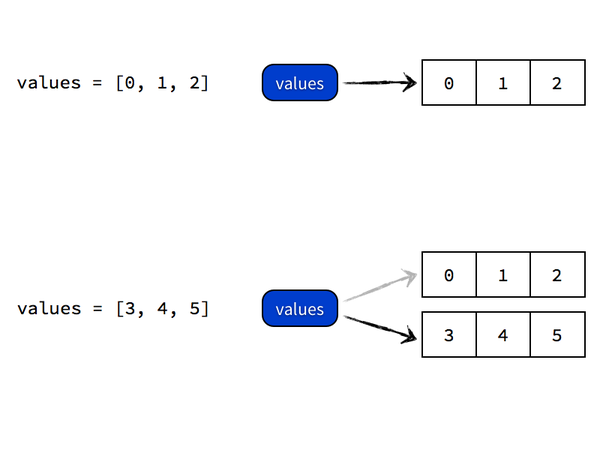执行
``values = values``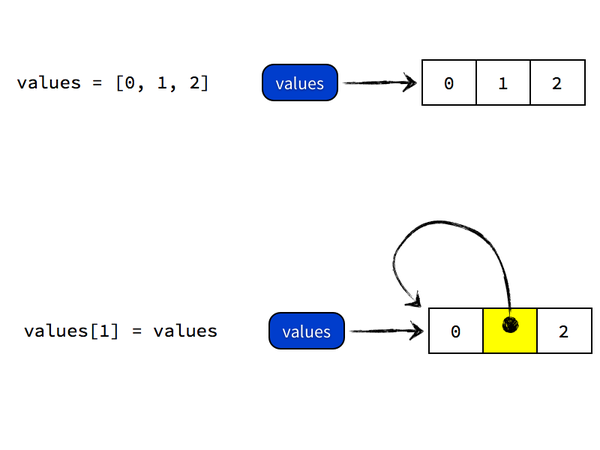``values[:]``

``values = values[:]``
Python 做的事情是，先 dereference 得到 values 所指向的对象 [0, 1, 2]，然后执行 [0, 1, 2][:] 复制操作得到一个新的对象，内容也是 [0, 1, 2]，然后将 values 所指向的列表对象的第二个元素指向这个复制二来的列表对象，最终 values 指向的对象是 [0, [0, 1, 2], 2]。过程如图所示：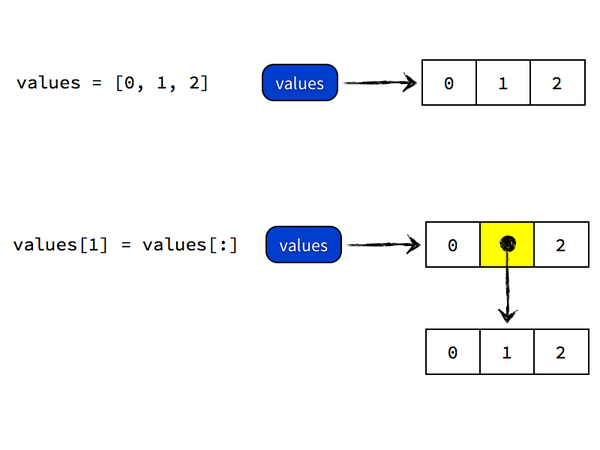往更深处说，values[:] 复制操作是所谓的「浅复制」(shallow copy)，当列表对象有嵌套的时候也会产生出乎意料的错误，比如
``a = [0, [1, 2], 3]b = a[:]a = 8a = 9``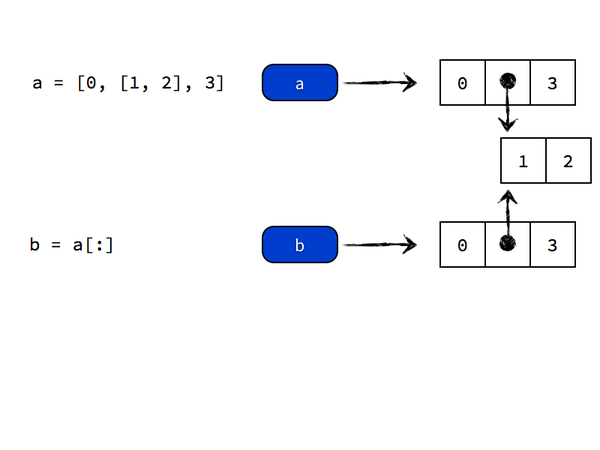正确的复制嵌套元素的方法是进行「深复制」(deep copy)，方法是
``import copya = [0, [1, 2], 3]b = copy.deepcopy(a)a = 8a = 9``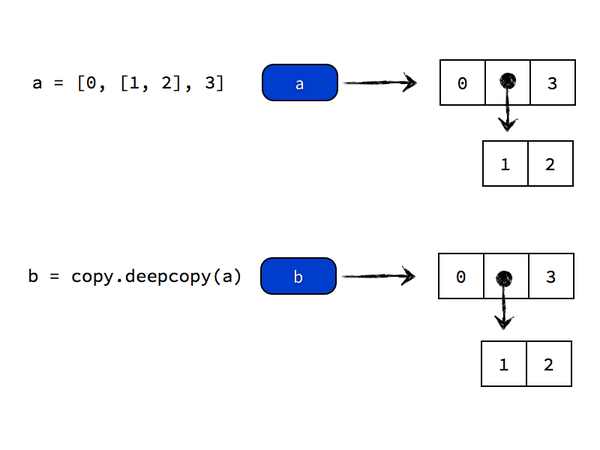`## first explain what is type="terms":  If type="terms" is selected, a matrix of predictions ## on the additive scale is produced, each column giving the deviations from the overall mean ## (of the original data's response, on the additive scale), which is given by the attribute "constant". set.seed(9999)x1=rnorm(10)x2=rnorm(10)y=rnorm(10)lmm=lm(y~x1+x2)predict(lmm, data=cbind(x1,x2,y), type="terms")   lmm\$coefficient+lmm\$coefficient*x1+mean(lmm\$coefficient*x2)-mean(y)-predlm[,1]lmm\$coefficient+lmm\$coefficient*x2+mean(lmm\$coefficient*x1)-mean(y)-predlm[,2]`

`library(ROCR)library(Hmisc) ## calculate AUC from the package ROCR and compare with it from Hmisc # method 1: from ROCRdata(ROCR.simple)pred=prediction(ROCR.simple\$prediction, ROCR.simple\$labels)perf=performance(pred, 'tpr', 'fpr')  #true positive and false negativeplot(perf, colorize=T) perf2=performance(pred, 'auc')auc=unlist(slot(perf2, 'y.values'))  # this is the AUC # method 2: from Hmiscrcorrstat=rcorr.cens(ROCR.simple\$prediction, ROCR.simple\$labels) rcorrstat  # 1st is AUC, 2nd is Accuracy Ratio(Gini Coefficient, or PowerStat, or Somer's D)`
Tagged with:

 123456789101112131415161718192021222324252627282930313233343536373839404142434445464748495051525354555657585960616263646566676869707172737475767778798081828384858687888990919293949596979899100101102103104105106107108109110111112113114115116117118119120121122123124125126127128129130131132133134135136137138139140141142143144145146147148149150151152153154155156157158159160161162163164165166167168169170171172173174175176177178179180181182183184185186187188189190191192193194195196197198199200201202203204205206207208209210211212213214215216217218219220221222223224225226227228229230231232233234235236237238239240241242243244245246247248249250251252253254255256257258259260261262263264265266267268269270271272273274275276277278279280281282283284285286287288289290291292293294295296297298299300301302303304305306307308309310311312313314315316317318319320321322323324325326327328329330331332333334335336337338339340341342343344345346347348349350351352353354355356357358359360361362363364365366367368369370371372373374375376377378379380381382383384385386387388389390391392393394395396397398399400401402403404405406407408409410411412413414415416417418419420 `### 1: R with Hadoop ###### there is a library called RHadoop by Revolutionary Analytics ###### http://www.lecturemaker.com/2011/02/rhipe/?doNavDotNow=14&lecID=1#video ##### 2: Run R job in linux serser #### R CMD BATCH rjob.R , then there will be generated .Rout file #### R --vanilla < myCode.R >& output.Rout & # source("rjob.R") to run from R console# R to execute system command: system function, and pipe to set up pipe connection# R system and foreign language interface: Sys.getenv, file.access, file.append, file.copy, # details: http://cran.r-project.org/doc/manuals/R-lang.html#System-and-foreign-language-interfaces# list objects in a package: ls("package:plyr")# list function in a package: lsf.str("package:plyr")# list data set in a package: data()# install.packages("plyr")### 3: R usrful commands ###attributes(mydata) names(mydata) class(mydata) scan, search(), data(), ls(), ls.str(), cat("\014") load attach, getwd() dir("/dir") setwd("/home/hsong"), options() history(max.show=Inf) savehistory() loadhistory()save.image() save(object) source() sink() apropos("sin"), gc(), system("ls -ltr")intersect, setdiff, union, unlink(".RData") # read in dataread.table, scan, cat# write to tablewrite.table(data_frame, file="./mydf", sep="/t", col.names=NA)save(df, file="./mydf"); load("./mydf")############### batch read in files ####################files=list.files(pattern=".txt\$")for (i in files){ x=read.table(i, header=T, row.names=1, comment.char="A", sep="\t") assign(i, x) write.table(x, paste(i, c(".out"), sep=""), quote=F, sep="\t", col.names=NA)}##########################################################sink("temp_R_output") ## redirect all subsequent output to temp_R_outputsink()### 3: R: do like SAS proc summary ###read.csv("D:/hsong/SkyDrive/Dropbox/sampledata.csv")->data1data.frame(data1)->data1attach(data1)names(data1) ### check column names of data frame in Raggregate(clicks,list(report_date,category_id),mean) aggregate(clicks~report_date+category_id, data1, mean)tapply(clicks,list(report_date,category_id),mean) #which is much faster### calculate the mean of clicks on report_date and category_id### similar to SAS: class report_date category_id; var clicks;### aggregate can handle multi vars while tapply can handle only one### 4: Interface from R to SAS ###system("C:\\Programe Files\\SAS\\SAS Foundation\\9.2\\SAS.exe        C:\\my_r_files\\test.sas"      )### Suppose test.sas is lke:     proc summary data=sashelp.class mean sum;      var height;      class sex;      output out=results;     run;          proc export data=results outfile="C:\tmp\results.csv" DBMS=csv replace;      putname=yes;     run;### Import the data to R:import_sas_data <- read.csv("C:\\tmp\results.csv", sep=",", header=T)### 5: calculate the statistics in subset and merge togetherlibrary(stats)aggregate(cbind(ncases, ncontrols) ~ alcgp + tobgp, data = esoph, sum)->data1aggregate(cbind(ncases, ncontrols) ~ alcgp , data = esoph, sum)->data2merge(data1, data2, by.x="alcgp", by.y="alcgp")### 6: R: pass parameters value from command line### vi rscript.R and input:args <- commandArgs(TRUE)x=as.numeric(args)xy=sin(x)y## Then go to the command line and run: Rscript rscript.R 1 2 3 4 5 6 7# the output will be:#  1 2 3 4 5 6 7#  0.8414710 0.9092974 0.1411200 -0.7568025 -0.9589243 -0.2794155 0.6569866### R: sort(x)=x[order(x)] ####### Calculate Frequency in R(function table) and SAS(proc freq) ###read.csv("sampledata.csv",header=T)->data1attach(data1)names(data1)table(PUBLISHER_ID,category_id)# then you can cite the output as a matrix #### 7: R: how to draw several graphs on one graph (overlap) ###after plot() draw the first one, use par(new=TRUE), then draw the second onesometimes it is better to use the same axis notations like all plots add the limitations as plot(¡­¡­¡­¡­,xlim=c))### 8: logistic regressionread.csv("D:\\hsong\\Desktop\\qcs.csv")->qsc1## pick three columns we wantedqsc=qsc1[,c("deals", "c_category_id", "payer_id")]attach(qsc)## change value in data.frame to factorsqsc\$c_category_id<-as.factor(c_category_id)qsc\$payer_id<-as.factor(payer_id)detach(qsc)## logistic regression and xs are factorsglm(deals~payer_id+c_category_id, family=binomial, data=qsc)## subset from data.frame by pick levels within factorssubset(qsc,payer_id %in% (550))->subset1### 9: usage of by: an example of weighted mean (it's better to use library(plyr))x=c( -0.14782, 0.62819, -1.73016, 0.04899, 0.48055, 1.52336, 0.76867, 0.29333, -1.18384, 0.96684, 0.50848, 2.25771, 0.14574, 0.50290, 0.26342)b=c(1:5,1:5,seq(1,9,by=2))f=rep(c(1,2,3),each=5)df=as.data.frame(cbind(x,b,f))by(df, list(df\$f), function(subset) weighted.mean(subset\$x, subset\$b))### use library(plyr)library(plyr)ddply(df,.(f), function(x) data.frame(wret=weighted.mean(x\$x,x\$b)))### 10: plot and then label each pointlibrary(foreign)read.spss("http://dl.dropbox.com/u/10684315/ucla_reg/crime.sav",to.data.frame=T)->crime## plot crime vs single and add state as the label to each pointplot(crime\$pctmetro,crime\$crime)text(crime\$pctmetro,crime\$crime,crime\$state,cex=.5,pos=2)### 11: add a new plot on the existed plotnames(crime)<-tolower(names(crime))row.names(crime)<-crime\$statelm(crime~pctmetro+pctsingle+poverty+single,crime)->crime_reg1library(car)dfbetas(crime_reg1)->dfb_reg1as.data.frame(dfb_reg1)->df_dfb_reg1plot(df_dfb_reg1\$pctmetro,col="red")par(new=T)plot(df_dfb_reg1\$single,col="green")### it can also be done by lines or points, which will draw graph in the existed plotplot(df_dfb_reg1\$pctmetro,col="red")points(df_dfb_reg1\$single,col="green")### 12: usful functions after linear regressionreg1=lm(y~x, data)plot(hatvalues(reg1),rstudent(reg1),cex=.5,col="red")cd=sqrt(cooks.distance(crime_reg1))vif(reg1)### 13: merge multiple dataframe(tables) together by multiple columnsDF1<-data.frame(var1=letters[1:5],a=rnorm(5), b=rnorm(5), c=rnorm(5))DF2<-data.frame(var1=letters[3:7],a=rnorm(5), b=rnorm(5), c=rnorm(5))DF3<-data.frame(var1=letters[6:10],a=rnorm(5), b=rnorm(5), c=rnorm(5))DF4<-data.frame(var1=letters[8:12],a=rnorm(5), b=rnorm(5), c=rnorm(5))DF<-DF1## this one not workfor (.df in (list(DF2, DF3, DF4))){ DF<-merge(DF,.df,by.x="var1",by.y="var1",all=T,suffixes=c("", ""))}## this one worksDF <- DF1for ( .df in list(DF2,DF3,DF4) ) { DF <-merge(DF,.df,by.x="var1", by.y="var1", all=T)        names(DF)[-1] <- paste(names(DF)[-1], 2:length(names(DF)))}names(DF) <- sub("[[:space:]].+\$", "", names(DF), perl=T)### 14: drop columns, date convert, aggregate and merge# remove all data in workspacerm(list=ls())# clear the screencat("\014")# read in data and treat . as missing(NA)read.csv("D:\\hsong\\SkyDrive\\r_temp\\visitnew_summary.csv",na.string='.',stringsAsFactors=F,header=T)->visnewhead(visnew)visnew\$report_date<-as.Date(visnew\$report_date,"%d-%b-%y")# check the class of each variable in the tablesapply(visnew,class)# lowcase all column namesnames(visnew)<-tolower(names(visnew))# drop two unnecessary columns, or use subset(visnew, select=-c(x_type_, x_freq_))visnew[,!(names(visnew) %in% c("x_type_", "x_freq_"))]->visnewshead(visnews)## aggregate dataaggregate(cbind(visits, revenue, clicks)~report_date,visnew,sum)->sum1## set the missing value as 0: visnew[is.na(visnew)]<-0 aggregate(cbind(visits, revenue, clicks)~report_date+publisher_id+category_id, visnew, sum)->sum2## be careful, if don't use na.rm, then the return value below will be NAaggregate(visnew[,c("visits","revenue","clicks")],by=list(rpt_dt=visnew\$report_date,pub_id=visnew\$publisher_id),sum,na.rm=T)## how to merge two df by multiple variablesdf29=sum2[sum2\$publisher_id==29,]df304=sum2[sum2\$publisher_id==304,]merge(df29,df304,by=c("report_date","category_id"))### 15: sort data# sorting examples using the mtcars datasetattach(mtcars)# sort by mpgnewdata <- mtcars[order(mpg),] # sort by mpg and cylnewdata <- mtcars[order(mpg, cyl),]#sort by mpg (ascending) and cyl (descending)newdata <- mtcars[order(mpg, -cyl),] detach(mtcars)### 16: subset of a data with subset funtion# pick the obs with cyl==4 and gear==4, and pick cols as belowsubset(mtcars, cyl==4 & gear==4, select=c("mpg", "cyl", "gear", "hp", "wt"))# drop the col hp and wtsubset(mtcars, select=-c(hp, wt))# pick the columns from mpg to amsubset(mtcars, select=mpg:am)### 17: use gl to generate factor levelsgl(n, k, length = n*k, labels = 1:n, ordered = FALSE)f1=gl(n=3,k=1,length=30, labels=c("A","B","C"))f2=gl(n=5,k=2,length=30, labels=1:5)### 18: Frequencies and Crosstabs: table, xtabs, ftable, crosstableattach(mtcars)tab1<-table(cyl, gear, carb)ftable(tab1)tab2<-xtabs(~cyl+gear+carb)ftable(tab2)detach(mtcars)### 19: regular expression# grep to capture the presence of a chargrep("apple", c("crab apple", "Apple jack", "apple sauce"))grep("apple", c("crab apple", "Apple jack", "apple sauce"), ignore.case=T)# strsplit to break string into small piecestext="Today is Monday, all the people are very happy to work"strsplit(text, ' ')# regexpr gregexpr to pinpint nad extract the position of a string in a regular expressionregexpr('[a-z]', c("today is monday", "007 is interesting", "2955 univ. dr", "apple store"))# replace and substituteprice=c("\$110,112.00", "\$3,567.23", "\$99,989.00", "1,028.98")sub('[\$,]','',price)# The two *sub functions differ only in that sub replaces only the first occurrence of a pattern whereas gsub replaces all occurrences.## compare to SAS a) paste("X", 1, sep='_') <-------> cat or ||  b) substring(state.name,1,2) <-------> substr(statename,1,2) c) strsplit(text, ' ') <-------> scan(text, 1, ' ') d) grep('pop', names(LifeCycleSavings)) <-------> index( , ) e) regexpr('[a-z][A-Z]', names(LifeCycleSavings)) <------->  f) gsub('[\$,]', '', "\$11,110.19") <-------> ### 20: migrate data from SAS to R# save sas data in transport formatlibname temp xport "/home/sas2r";data temp.mydata; set my_sas_data;run;# in R, read in the data with sasxport.get function from library(Hmisc)library(Hmisc)mydata<-sasxport.get("/home/sas2r")# to export the data to an txt file, delimited by Tabwrite.table(mydata, "/home/rout", sep="\t")### 21: reshape data from long to wide or wide to long with library(reshape2)aggregate(cbind(mpg, wt)~gear+carb, mtcars, sum)->agg1library(reshape2)# from wide to longmelt(agg1, id.var=c("gear","carb"), measure.var=c("mpg", "wt"))->w2l# from long to widedcast(w2l, gear+carb~variable, value.var="value")## be careful when use dcast, the values of gear and carb should be unique. if not, then it will issue warnning like "Aggregation function missing: defaulting to length" because this dcast will do aggregate for melt result.### 22: replicate the job of PROC RANK in SAStable(cut(lprpv, breaks=quantile(lprpv, probs=c(0:20/20)), labels=0:19, include.lowest=T, right=F))table(cut(cars\$speed, breaks=quantile(cars\$speed, probs=c(0:15/15)), labels=1:15, include.lowest=TRUE))### 23: in R run the linux system commandsystem("ls -ltr")### 24: variable selection with step function# R provides the step function to do variable selection by AIC# variable selection cannot solve the multicollinearity issuelm1=lm(y~x+x1+x2+x3+x4+x5)summary(lm1)step(lm1)### 25: batch import and export many filesfiles<-list.files(pattern="*.txt\$")for (i in files){ x<-read.table(i, header=T, comment.char="A", sep="\t") assign(i, x) print(i) write.table(x,paste(i, c(".out"), sep=""), quote=F, sep="\t", col.names=NA)}### 26: loops in R# 1: for loopsx=c("one", "two", "three")for (i in x){ print (i)}# 2: while loopx=1while (x<=10){ print(x) x=x+2}# 3: repeat loopx=1repeat{ x=x+2 if (x>=10) break() print(x)}# 4: apply, sapply, lapply, tapplyx=matrix(1:80,8,10, byrow=T)apply(x,1,sum) # apply(X, MARGIN, FUN, ARGs)### 27: use scan to read in temp data for ad-hoc analysis# like cp data from sas proc print to draw graph in R. We can save data in txt then read.table.# a simple way is to copy and paste to scan functionx=scan(what=list(a1=0, a2=0))# then paste the copied data(two columns)y=data.frame(x)### 28: read in SAS data set into R (SAS is necessary)##1: first use SAS to export data to csv or dlm, then use Rlibname test "/data02/temp/temp_hsong/test";proc export data=test.hsb12 outfile="/data02/temp/temp_hsong/test/sasxport" dbms=dlm replace;        delimiter=",";run;# Then read in the exported data with Rread.table("/data02/temp/temp_hsong/test/sasxport.txt", header=T)##2: to read in with the package {Hmisc}library(Hmisc)hsb12=sas.get(lib="/data02/temp/temp_hsong/test", mem="hsb12", as.is=T)##3: read with library {foreign}, but I did not run it successfullyread.xport("path")### 29: R: tips and tricks to increase the reading speed# test to read /data02/temp/temp_hsong/test/hsb12.sas7bdatsystem.time(read.table("/data02/temp/temp_hsong/test/sasxport.txt", header=T))system.time(read.table("/data02/temp/temp_hsong/test/sasxport.txt", header=T, stringsAsFactors=F))system.time(read.table("/data02/temp/temp_hsong/test/sasxport.txt", header=T, comment.char=''))system.time(read.table("/data02/temp/temp_hsong/test/sasxport.txt", header=T, nrows=8000))system.time(read.table("/data02/temp/temp_hsong/test/sasxport.txt", header=T, sep=',', colClasses=c(rep("numeric",11))))### 30: R in piecewise linear model, an example from r-bloggersN <- 40 # number of sampled pointsK <- 5 # number of knotspiece.formula <- function(var.name, knots) { formula.sign <- rep(" - ", length(knots))     formula.sign[knots < 0] <- " + " paste(var.name, "+",              paste("I(pmax(", var.name, formula.sign, abs(knots), ", 0))", collapse = " + ", sep=""))}f <- function(x) {    2 * sin(6 * x)}set.seed(1)x <- seq(-1, 1, len = N)y <- f(x) + rnorm(length(x))knots <- seq(min(x), max(x), len = K + 2)[-c(1, K + 2)]model <- lm(formula(paste("y ~", piece.formula("x", knots))))par(mar = c(4, 4, 1, 1))plot(x, y)lines(x, f(x))new.x <- seq(min(x), max(x) ,len = 10000)points(new.x, predict(model, newdata = data.frame(x = new.x)), col = "red", pch = ".")points(knots, predict(model, newdata = data.frame(x = knots)), col = "red", pch = 18)### 31: R: check what a function is rather than what a function does# method 1: use :::methods(kruskal.test)stats:::kruskal.test.default# method 2: use getAnywheremethods(krustal.test)getAnywhere(krustal.test.default)# method 3: go the the R source code, name.tar.gz filefind the .tar.gz version of the package, look for the 'R' directory and you will find the source code (with the developper's comments, if any)`

 123456789101112131415161718192021222324252627282930313233343536373839404142434445464748495051525354555657585960616263646566676869707172737475767778798081828384858687888990919293949596979899100101102103104105106107108109110111112113114115116117118119120121122123124125126127128129130131132133134135136137138139140141142143144145146147148149150151152153154155156157158159160161162163164165166167168169170171172173174175176177178179180181182183184185186187188189190191192193194195196197198199200201202203204205206207208209210211212213214215216217218219220221222223224225226227228229230231232233234235236237238239240241242243244245246247248249250251252253254255256257258259260261262263264265266267268269270271272273274275276277278279280281282283284285286287288289290291292293294295296297298299300301302303304305306307308309310311312313314315316317318319320321322323324325326327328329330331332333334335336337338339340341342343344345346347348349350351352353354355356357358359360361362363364365366367368369370371372373374375376377378379380381382383384385386387388389390391392393394395396397398399400401402403404405406407408409410411412413414415416417418419420 `### 1: R with Hadoop ###### there is a library called RHadoop by Revolutionary Analytics ###### http://www.lecturemaker.com/2011/02/rhipe/?doNavDotNow=14&lecID=1#video ##### 2: Run R job in linux serser #### R CMD BATCH rjob.R , then there will be generated .Rout file #### R --vanilla < myCode.R >& output.Rout & # source("rjob.R") to run from R console# R to execute system command: system function, and pipe to set up pipe connection# R system and foreign language interface: Sys.getenv, file.access, file.append, file.copy, # details: http://cran.r-project.org/doc/manuals/R-lang.html#System-and-foreign-language-interfaces# list objects in a package: ls("package:plyr")# list function in a package: lsf.str("package:plyr")# list data set in a package: data()# install.packages("plyr")### 3: R usrful commands ###attributes(mydata) names(mydata) class(mydata) scan, search(), data(), ls(), ls.str(), cat("\014") load attach, getwd() dir("/dir") setwd("/home/hsong"), options() history(max.show=Inf) savehistory() loadhistory()save.image() save(object) source() sink() apropos("sin"), gc(), system("ls -ltr")intersect, setdiff, union, unlink(".RData") # read in dataread.table, scan, cat# write to tablewrite.table(data_frame, file="./mydf", sep="/t", col.names=NA)save(df, file="./mydf"); load("./mydf")############### batch read in files ####################files=list.files(pattern=".txt\$")for (i in files){ x=read.table(i, header=T, row.names=1, comment.char="A", sep="\t") assign(i, x) write.table(x, paste(i, c(".out"), sep=""), quote=F, sep="\t", col.names=NA)}##########################################################sink("temp_R_output") ## redirect all subsequent output to temp_R_outputsink()### 3: R: do like SAS proc summary ###read.csv("D:/hsong/SkyDrive/Dropbox/sampledata.csv")->data1data.frame(data1)->data1attach(data1)names(data1) ### check column names of data frame in Raggregate(clicks,list(report_date,category_id),mean) aggregate(clicks~report_date+category_id, data1, mean)tapply(clicks,list(report_date,category_id),mean) #which is much faster### calculate the mean of clicks on report_date and category_id### similar to SAS: class report_date category_id; var clicks;### aggregate can handle multi vars while tapply can handle only one### 4: Interface from R to SAS ###system("C:\\Programe Files\\SAS\\SAS Foundation\\9.2\\SAS.exe        C:\\my_r_files\\test.sas"      )### Suppose test.sas is lke:     proc summary data=sashelp.class mean sum;      var height;      class sex;      output out=results;     run;          proc export data=results outfile="C:\tmp\results.csv" DBMS=csv replace;      putname=yes;     run;### Import the data to R:import_sas_data <- read.csv("C:\\tmp\results.csv", sep=",", header=T)### 5: calculate the statistics in subset and merge togetherlibrary(stats)aggregate(cbind(ncases, ncontrols) ~ alcgp + tobgp, data = esoph, sum)->data1aggregate(cbind(ncases, ncontrols) ~ alcgp , data = esoph, sum)->data2merge(data1, data2, by.x="alcgp", by.y="alcgp")### 6: R: pass parameters value from command line### vi rscript.R and input:args <- commandArgs(TRUE)x=as.numeric(args)xy=sin(x)y## Then go to the command line and run: Rscript rscript.R 1 2 3 4 5 6 7# the output will be:#  1 2 3 4 5 6 7#  0.8414710 0.9092974 0.1411200 -0.7568025 -0.9589243 -0.2794155 0.6569866### R: sort(x)=x[order(x)] ####### Calculate Frequency in R(function table) and SAS(proc freq) ###read.csv("sampledata.csv",header=T)->data1attach(data1)names(data1)table(PUBLISHER_ID,category_id)# then you can cite the output as a matrix #### 7: R: how to draw several graphs on one graph (overlap) ###after plot() draw the first one, use par(new=TRUE), then draw the second onesometimes it is better to use the same axis notations like all plots add the limitations as plot(¡­¡­¡­¡­,xlim=c))### 8: logistic regressionread.csv("D:\\hsong\\Desktop\\qcs.csv")->qsc1## pick three columns we wantedqsc=qsc1[,c("deals", "c_category_id", "payer_id")]attach(qsc)## change value in data.frame to factorsqsc\$c_category_id<-as.factor(c_category_id)qsc\$payer_id<-as.factor(payer_id)detach(qsc)## logistic regression and xs are factorsglm(deals~payer_id+c_category_id, family=binomial, data=qsc)## subset from data.frame by pick levels within factorssubset(qsc,payer_id %in% (550))->subset1### 9: usage of by: an example of weighted mean (it's better to use library(plyr))x=c( -0.14782, 0.62819, -1.73016, 0.04899, 0.48055, 1.52336, 0.76867, 0.29333, -1.18384, 0.96684, 0.50848, 2.25771, 0.14574, 0.50290, 0.26342)b=c(1:5,1:5,seq(1,9,by=2))f=rep(c(1,2,3),each=5)df=as.data.frame(cbind(x,b,f))by(df, list(df\$f), function(subset) weighted.mean(subset\$x, subset\$b))### use library(plyr)library(plyr)ddply(df,.(f), function(x) data.frame(wret=weighted.mean(x\$x,x\$b)))### 10: plot and then label each pointlibrary(foreign)read.spss("http://dl.dropbox.com/u/10684315/ucla_reg/crime.sav",to.data.frame=T)->crime## plot crime vs single and add state as the label to each pointplot(crime\$pctmetro,crime\$crime)text(crime\$pctmetro,crime\$crime,crime\$state,cex=.5,pos=2)### 11: add a new plot on the existed plotnames(crime)<-tolower(names(crime))row.names(crime)<-crime\$statelm(crime~pctmetro+pctsingle+poverty+single,crime)->crime_reg1library(car)dfbetas(crime_reg1)->dfb_reg1as.data.frame(dfb_reg1)->df_dfb_reg1plot(df_dfb_reg1\$pctmetro,col="red")par(new=T)plot(df_dfb_reg1\$single,col="green")### it can also be done by lines or points, which will draw graph in the existed plotplot(df_dfb_reg1\$pctmetro,col="red")points(df_dfb_reg1\$single,col="green")### 12: usful functions after linear regressionreg1=lm(y~x, data)plot(hatvalues(reg1),rstudent(reg1),cex=.5,col="red")cd=sqrt(cooks.distance(crime_reg1))vif(reg1)### 13: merge multiple dataframe(tables) together by multiple columnsDF1<-data.frame(var1=letters[1:5],a=rnorm(5), b=rnorm(5), c=rnorm(5))DF2<-data.frame(var1=letters[3:7],a=rnorm(5), b=rnorm(5), c=rnorm(5))DF3<-data.frame(var1=letters[6:10],a=rnorm(5), b=rnorm(5), c=rnorm(5))DF4<-data.frame(var1=letters[8:12],a=rnorm(5), b=rnorm(5), c=rnorm(5))DF<-DF1## this one not workfor (.df in (list(DF2, DF3, DF4))){ DF<-merge(DF,.df,by.x="var1",by.y="var1",all=T,suffixes=c("", ""))}## this one worksDF <- DF1for ( .df in list(DF2,DF3,DF4) ) { DF <-merge(DF,.df,by.x="var1", by.y="var1", all=T)        names(DF)[-1] <- paste(names(DF)[-1], 2:length(names(DF)))}names(DF) <- sub("[[:space:]].+\$", "", names(DF), perl=T)### 14: drop columns, date convert, aggregate and merge# remove all data in workspacerm(list=ls())# clear the screencat("\014")# read in data and treat . as missing(NA)read.csv("D:\\hsong\\SkyDrive\\r_temp\\visitnew_summary.csv",na.string='.',stringsAsFactors=F,header=T)->visnewhead(visnew)visnew\$report_date<-as.Date(visnew\$report_date,"%d-%b-%y")# check the class of each variable in the tablesapply(visnew,class)# lowcase all column namesnames(visnew)<-tolower(names(visnew))# drop two unnecessary columns, or use subset(visnew, select=-c(x_type_, x_freq_))visnew[,!(names(visnew) %in% c("x_type_", "x_freq_"))]->visnewshead(visnews)## aggregate dataaggregate(cbind(visits, revenue, clicks)~report_date,visnew,sum)->sum1## set the missing value as 0: visnew[is.na(visnew)]<-0 aggregate(cbind(visits, revenue, clicks)~report_date+publisher_id+category_id, visnew, sum)->sum2## be careful, if don't use na.rm, then the return value below will be NAaggregate(visnew[,c("visits","revenue","clicks")],by=list(rpt_dt=visnew\$report_date,pub_id=visnew\$publisher_id),sum,na.rm=T)## how to merge two df by multiple variablesdf29=sum2[sum2\$publisher_id==29,]df304=sum2[sum2\$publisher_id==304,]merge(df29,df304,by=c("report_date","category_id"))### 15: sort data# sorting examples using the mtcars datasetattach(mtcars)# sort by mpgnewdata <- mtcars[order(mpg),] # sort by mpg and cylnewdata <- mtcars[order(mpg, cyl),]#sort by mpg (ascending) and cyl (descending)newdata <- mtcars[order(mpg, -cyl),] detach(mtcars)### 16: subset of a data with subset funtion# pick the obs with cyl==4 and gear==4, and pick cols as belowsubset(mtcars, cyl==4 & gear==4, select=c("mpg", "cyl", "gear", "hp", "wt"))# drop the col hp and wtsubset(mtcars, select=-c(hp, wt))# pick the columns from mpg to amsubset(mtcars, select=mpg:am)### 17: use gl to generate factor levelsgl(n, k, length = n*k, labels = 1:n, ordered = FALSE)f1=gl(n=3,k=1,length=30, labels=c("A","B","C"))f2=gl(n=5,k=2,length=30, labels=1:5)### 18: Frequencies and Crosstabs: table, xtabs, ftable, crosstableattach(mtcars)tab1<-table(cyl, gear, carb)ftable(tab1)tab2<-xtabs(~cyl+gear+carb)ftable(tab2)detach(mtcars)### 19: regular expression# grep to capture the presence of a chargrep("apple", c("crab apple", "Apple jack", "apple sauce"))grep("apple", c("crab apple", "Apple jack", "apple sauce"), ignore.case=T)# strsplit to break string into small piecestext="Today is Monday, all the people are very happy to work"strsplit(text, ' ')# regexpr gregexpr to pinpint nad extract the position of a string in a regular expressionregexpr('[a-z]', c("today is monday", "007 is interesting", "2955 univ. dr", "apple store"))# replace and substituteprice=c("\$110,112.00", "\$3,567.23", "\$99,989.00", "1,028.98")sub('[\$,]','',price)# The two *sub functions differ only in that sub replaces only the first occurrence of a pattern whereas gsub replaces all occurrences.## compare to SAS a) paste("X", 1, sep='_') <-------> cat or ||  b) substring(state.name,1,2) <-------> substr(statename,1,2) c) strsplit(text, ' ') <-------> scan(text, 1, ' ') d) grep('pop', names(LifeCycleSavings)) <-------> index( , ) e) regexpr('[a-z][A-Z]', names(LifeCycleSavings)) <------->  f) gsub('[\$,]', '', "\$11,110.19") <-------> ### 20: migrate data from SAS to R# save sas data in transport formatlibname temp xport "/home/sas2r";data temp.mydata; set my_sas_data;run;# in R, read in the data with sasxport.get function from library(Hmisc)library(Hmisc)mydata<-sasxport.get("/home/sas2r")# to export the data to an txt file, delimited by Tabwrite.table(mydata, "/home/rout", sep="\t")### 21: reshape data from long to wide or wide to long with library(reshape2)aggregate(cbind(mpg, wt)~gear+carb, mtcars, sum)->agg1library(reshape2)# from wide to longmelt(agg1, id.var=c("gear","carb"), measure.var=c("mpg", "wt"))->w2l# from long to widedcast(w2l, gear+carb~variable, value.var="value")## be careful when use dcast, the values of gear and carb should be unique. if not, then it will issue warnning like "Aggregation function missing: defaulting to length" because this dcast will do aggregate for melt result.### 22: replicate the job of PROC RANK in SAStable(cut(lprpv, breaks=quantile(lprpv, probs=c(0:20/20)), labels=0:19, include.lowest=T, right=F))table(cut(cars\$speed, breaks=quantile(cars\$speed, probs=c(0:15/15)), labels=1:15, include.lowest=TRUE))### 23: in R run the linux system commandsystem("ls -ltr")### 24: variable selection with step function# R provides the step function to do variable selection by AIC# variable selection cannot solve the multicollinearity issuelm1=lm(y~x+x1+x2+x3+x4+x5)summary(lm1)step(lm1)### 25: batch import and export many filesfiles<-list.files(pattern="*.txt\$")for (i in files){ x<-read.table(i, header=T, comment.char="A", sep="\t") assign(i, x) print(i) write.table(x,paste(i, c(".out"), sep=""), quote=F, sep="\t", col.names=NA)}### 26: loops in R# 1: for loopsx=c("one", "two", "three")for (i in x){ print (i)}# 2: while loopx=1while (x<=10){ print(x) x=x+2}# 3: repeat loopx=1repeat{ x=x+2 if (x>=10) break() print(x)}# 4: apply, sapply, lapply, tapplyx=matrix(1:80,8,10, byrow=T)apply(x,1,sum) # apply(X, MARGIN, FUN, ARGs)### 27: use scan to read in temp data for ad-hoc analysis# like cp data from sas proc print to draw graph in R. We can save data in txt then read.table.# a simple way is to copy and paste to scan functionx=scan(what=list(a1=0, a2=0))# then paste the copied data(two columns)y=data.frame(x)### 28: read in SAS data set into R (SAS is necessary)##1: first use SAS to export data to csv or dlm, then use Rlibname test "/data02/temp/temp_hsong/test";proc export data=test.hsb12 outfile="/data02/temp/temp_hsong/test/sasxport" dbms=dlm replace;        delimiter=",";run;# Then read in the exported data with Rread.table("/data02/temp/temp_hsong/test/sasxport.txt", header=T)##2: to read in with the package {Hmisc}library(Hmisc)hsb12=sas.get(lib="/data02/temp/temp_hsong/test", mem="hsb12", as.is=T)##3: read with library {foreign}, but I did not run it successfullyread.xport("path")### 29: R: tips and tricks to increase the reading speed# test to read /data02/temp/temp_hsong/test/hsb12.sas7bdatsystem.time(read.table("/data02/temp/temp_hsong/test/sasxport.txt", header=T))system.time(read.table("/data02/temp/temp_hsong/test/sasxport.txt", header=T, stringsAsFactors=F))system.time(read.table("/data02/temp/temp_hsong/test/sasxport.txt", header=T, comment.char=''))system.time(read.table("/data02/temp/temp_hsong/test/sasxport.txt", header=T, nrows=8000))system.time(read.table("/data02/temp/temp_hsong/test/sasxport.txt", header=T, sep=',', colClasses=c(rep("numeric",11))))### 30: R in piecewise linear model, an example from r-bloggersN <- 40 # number of sampled pointsK <- 5 # number of knotspiece.formula <- function(var.name, knots) { formula.sign <- rep(" - ", length(knots))     formula.sign[knots < 0] <- " + " paste(var.name, "+",              paste("I(pmax(", var.name, formula.sign, abs(knots), ", 0))", collapse = " + ", sep=""))}f <- function(x) {    2 * sin(6 * x)}set.seed(1)x <- seq(-1, 1, len = N)y <- f(x) + rnorm(length(x))knots <- seq(min(x), max(x), len = K + 2)[-c(1, K + 2)]model <- lm(formula(paste("y ~", piece.formula("x", knots))))par(mar = c(4, 4, 1, 1))plot(x, y)lines(x, f(x))new.x <- seq(min(x), max(x) ,len = 10000)points(new.x, predict(model, newdata = data.frame(x = new.x)), col = "red", pch = ".")points(knots, predict(model, newdata = data.frame(x = knots)), col = "red", pch = 18)### 31: R: check what a function is rather than what a function does# method 1: use :::methods(kruskal.test)stats:::kruskal.test.default# method 2: use getAnywheremethods(krustal.test)getAnywhere(krustal.test.default)# method 3: go the the R source code, name.tar.gz filefind the .tar.gz version of the package, look for the 'R' directory and you will find the source code (with the developper's comments, if any)`

learn proc format:

``data test;input start \$ end \$ label;cards;nextag   nextag 1#sina    #sina  2#1000    #1000  3;run;data fmt0;retain fmtname'\$testfmt' ;set test end=last;start=start;end=end;label=label;output;if last then do;  hlo='O';  label=0;  output;end;run;proc print data=fmt0;run;proc format cntlin=fmt0;select \$testfmt;run;data test;input test \$20.;cards;nextag#sina&ok#1000wokong;run;data test_result;set test;id=put(test, \$testfmt.)+0;run;proc print data=test_result;run;``

The output is:

``Obs    test      id 1     nextag     1 2     #sina      2 3     &ok        0 4     #1000      3 5     wokong     0``

A new data set test1 is read from test(two variables: id, a). We want to create an index for test1(index name is called aid since aid is indexed in data set aid). Pay attention how index, rename and keep is used in data step test1.

``data test; input id a; cards; 1 1 3 3 2 5 ;run;data aid (index=(aid)); input aid b; cards; 1 1 2 3 3 4 ;run;data test1(index=(aid)); set test(keep=id a ); rename id =  aid;run;proc contents data=test1;run;data merge_1; merge aid test1; by aid;run;proc print data=merge_1;run;``

The question is: after calculate the percentage, we want to count from how many obs are in 0-1%, how many in 1%-2%, ..., how many in 99%-100%. For this simple example, we can use round or floor or ceil to get the result. But here shows how to use proc format to get it.

After we get cumulative percentage, we format the percentage with put function. Take care in the proc format don't forget to add hlo, otherwise it will gives error since the first start number is missing.

``data test; do i=1 to 1000;   x=ranpoi(8,8);   output; end;run;data test; set test; y+x;run;proc sql; create table test as select x, y, y/max(y) as pct from test;quit;data test2; do i=1 to 100;   x=i/100;   y=lag(x);   output; end;run;data a; set test2; fmtname='fmttestf'; start=y; end=x; label=i; eexcl='Y'; if _n_=1 then hlo='L';run;proc format cntlin=a;select fmttestf;run;data final; set test; rank=put(pct, fmttestf.)+0;run;proc print data=final;run;proc sql; select rank, count(1) as cnt from final group by rank order by rank;quit;``